# 三维薄结构热传导问题分层二次元方程的多水平方法*

(湘潭大学 土木工程与力学学院， 湖南 湘潭 411105)

## 1 模型问题及分层有限元离散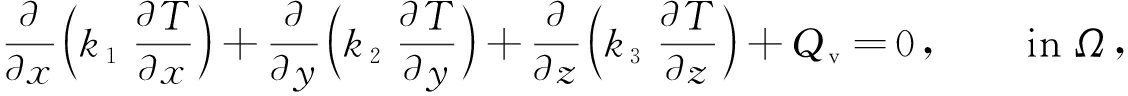(1)

T=TΓ, on Γ1,

(2)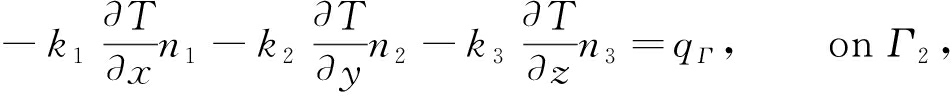(3)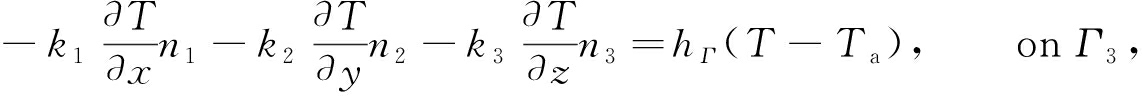(4)

a(T,W)=F(W),

(5)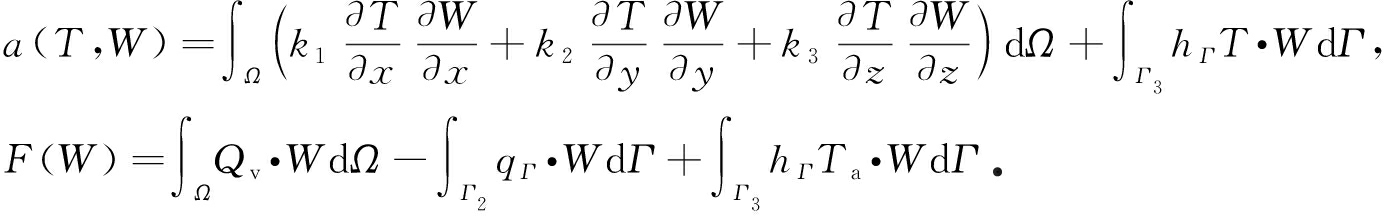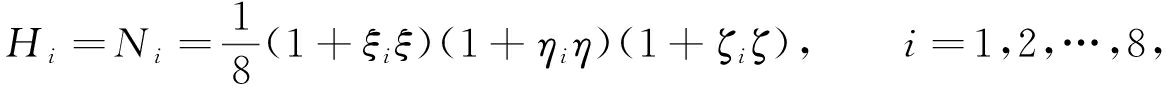(6)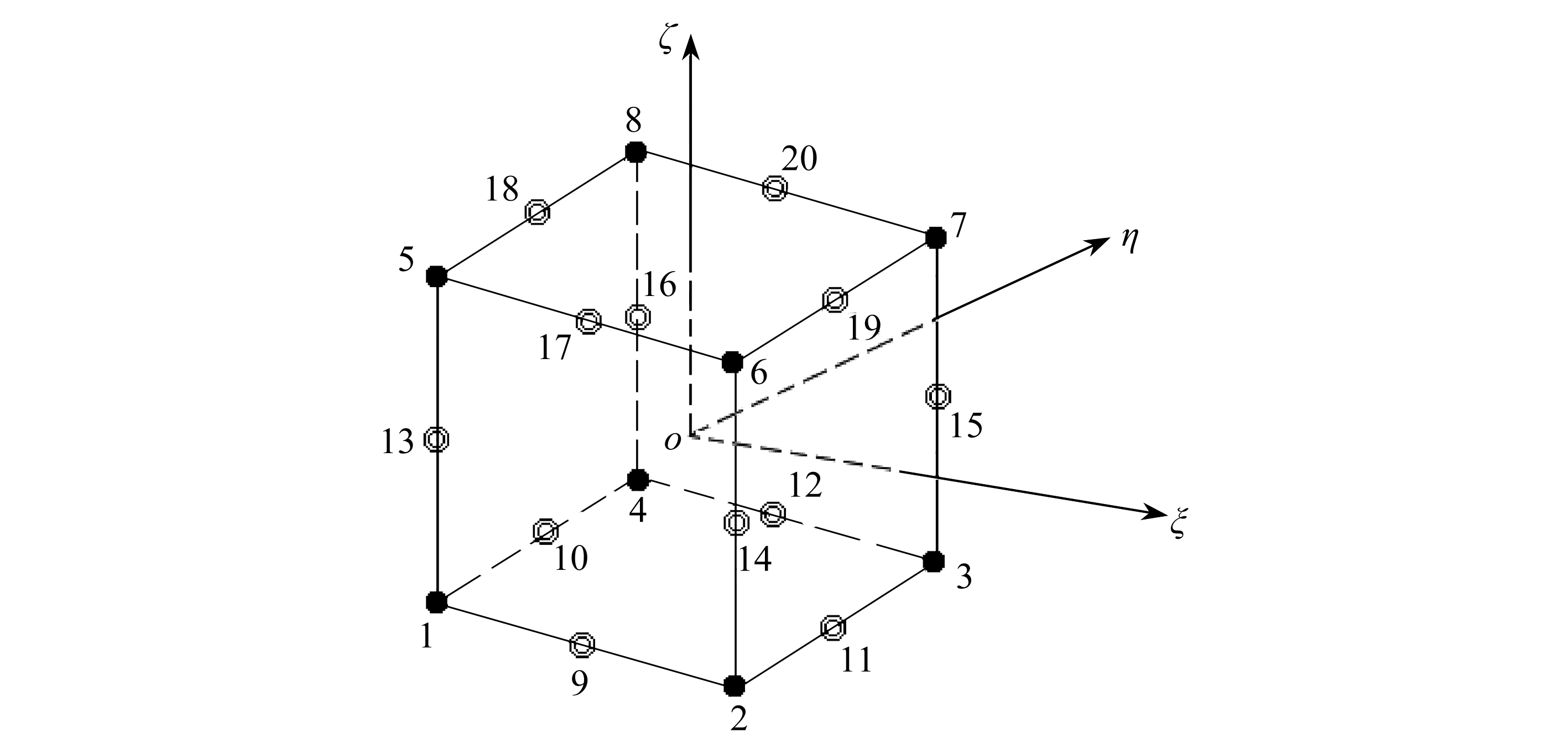Fig. 1 The 20-node reference element in the local coordinate system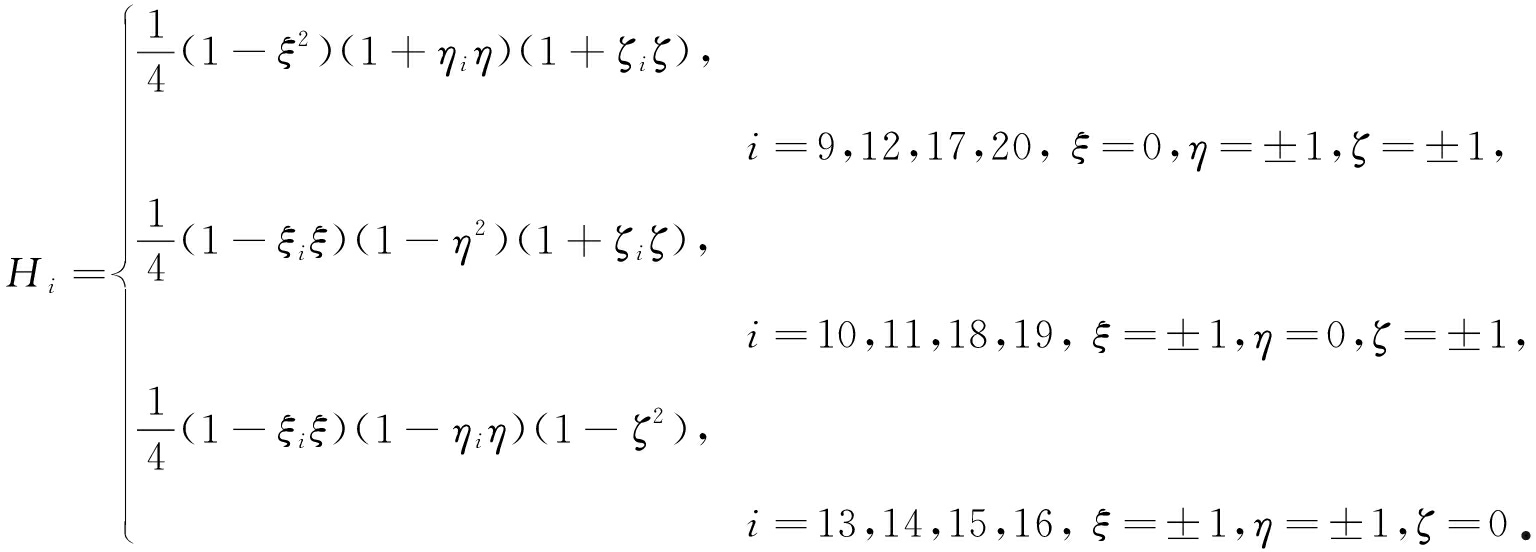Kq=p

(7)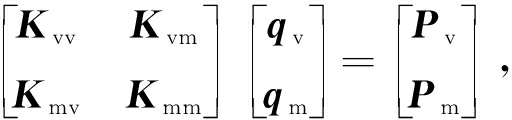(8)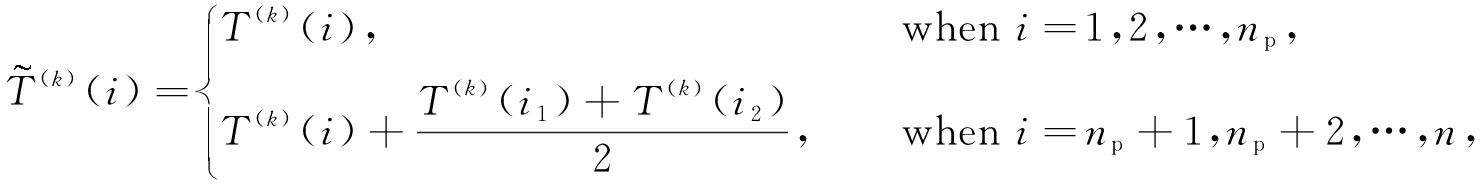(9)

Table 1 Condition numbers of 2 matrices K and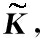and the iteration counts of the CG method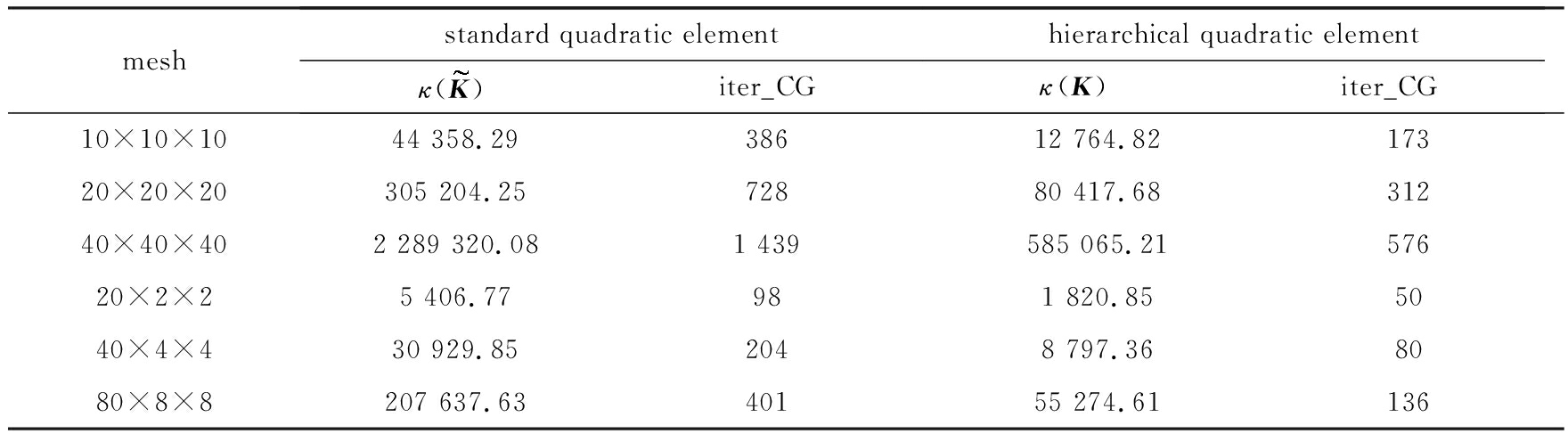## 2 分层二次元方程的多水平方法

### 2.1两水平方法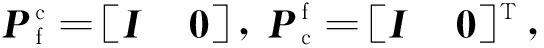(10)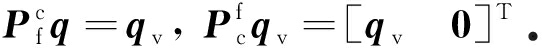(11)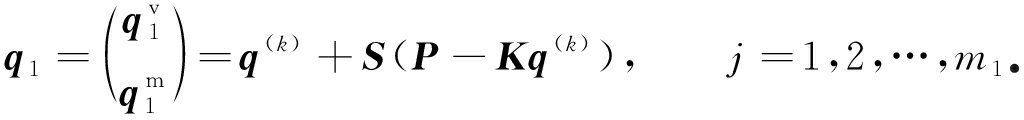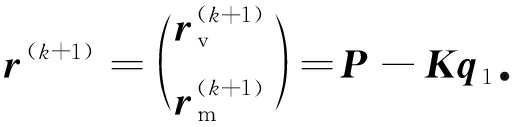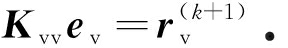(12)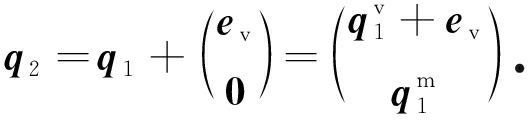q(k+1)=q2+ST(P-Kq2)， j=1,2,…,m2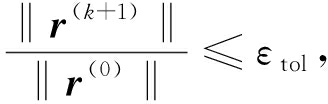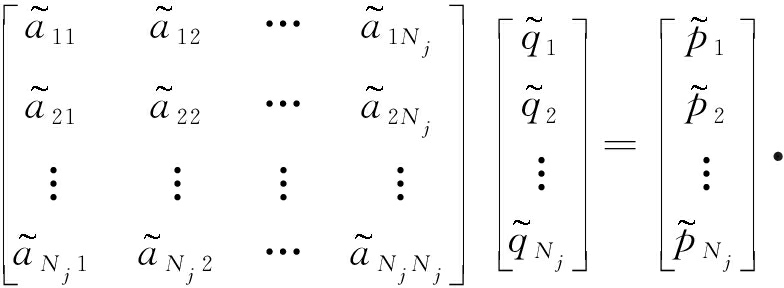q=(q1,q2,…,qn)T

① LBGS_v smoother：仅对所有顶点，在其patches上做一次LBGS迭代；

② LBGS_m smoother：仅对所有边中点，在其patches 上做一次LBGS迭代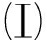常系数：

k1=15.0 W/(m2·℃)， k2=10.0 W/(m2·℃)， k3=5.0 W/(m2·℃)， Qv=0 W/m2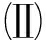变系数：

k1=25x2-10y-33z+4， k2=20x-50y2+12z+3， k3=10x+48y+5z2+2，

Qv=1/((x+0.001)(y+0.01)(z+0.01))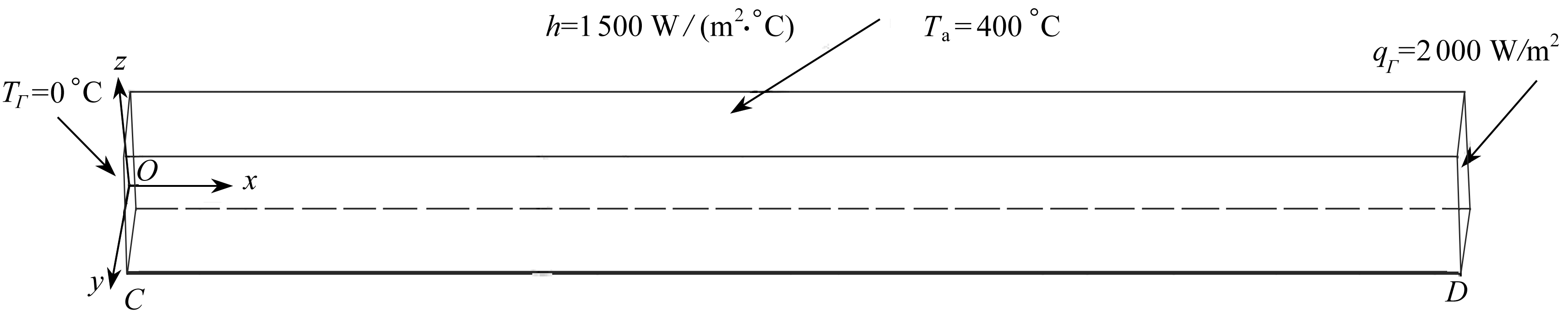Fig. 2 The geometry structure of the conduction beam considered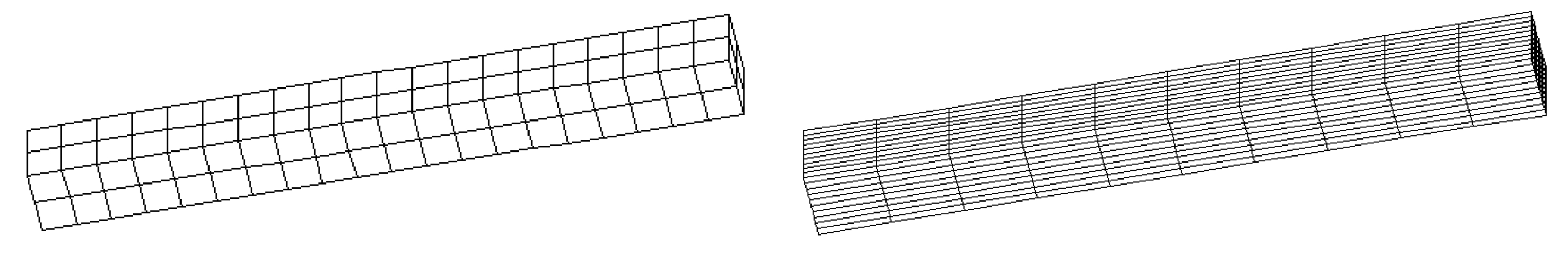(a) 各向同性网格(20×2×2) (b) 各向异性网格(10×10×10)
(a) The isotropic hexahedral mesh with 20×2×2 elements (b) The anisotropic hexahedral mesh with 10×10×10 elements

Fig. 3 Two types of meshes used for the conduction beam

Table 2 Iteration counts of the HTL methods for the hierarchical quadratic discretizations of the conduction beam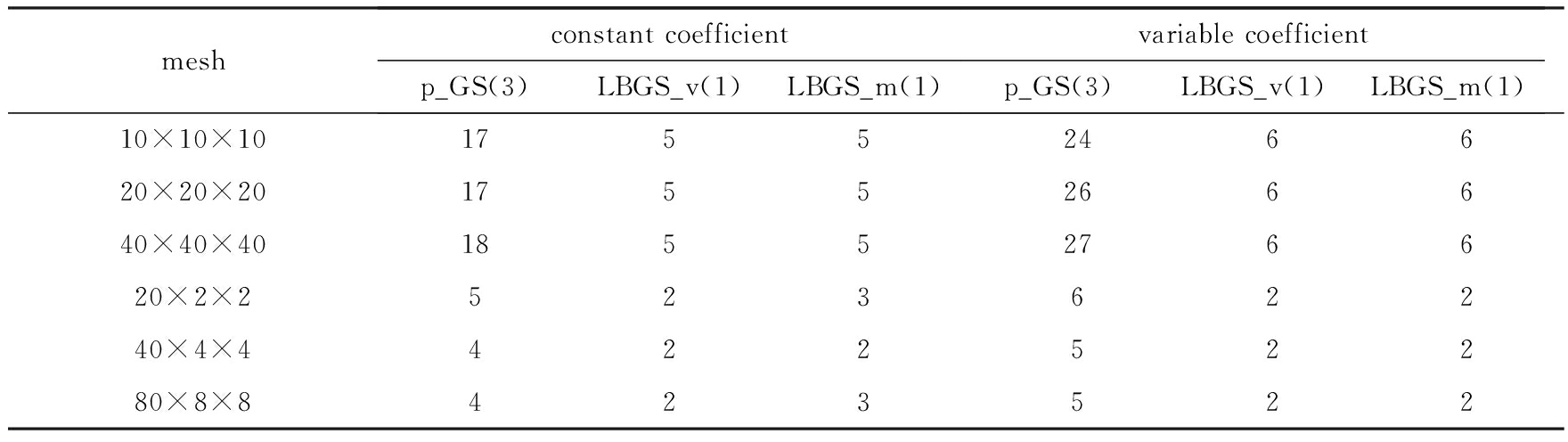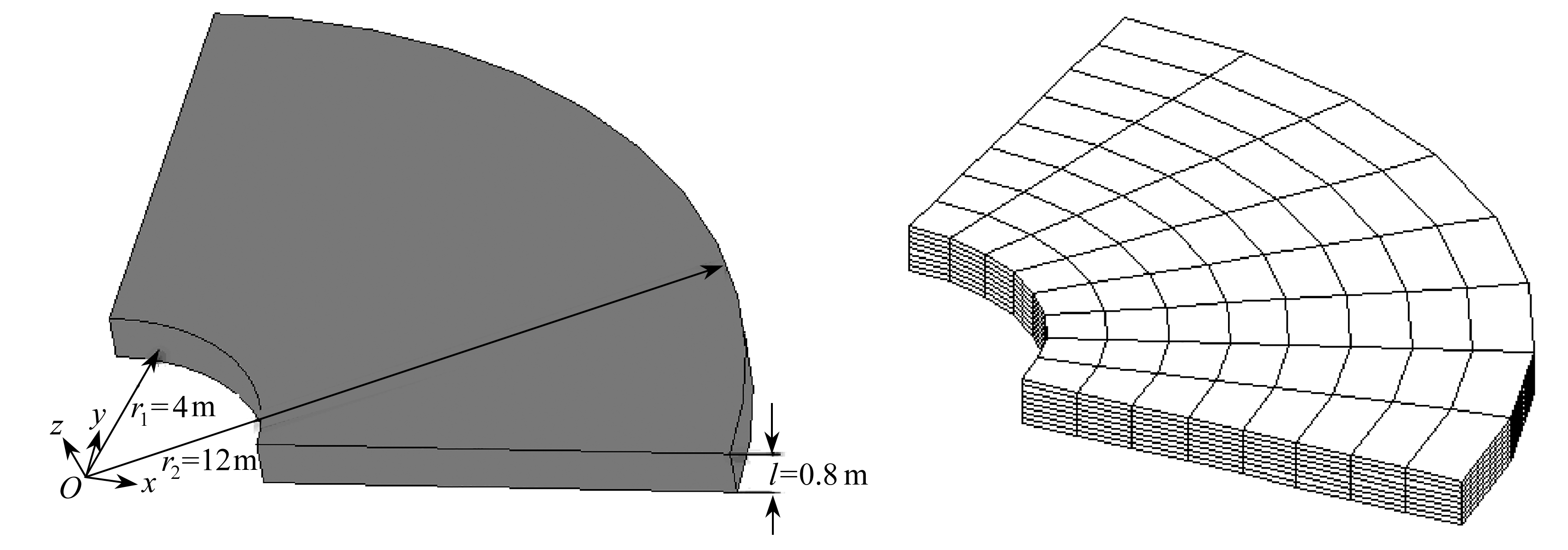Fig. 4 The geometry structure of the thin-walled sector and one sample mesh (8×8×8)

Table 3 Iteration counts of the HTL methods for the hierarchical quadratic discretizations of the thin-walled sector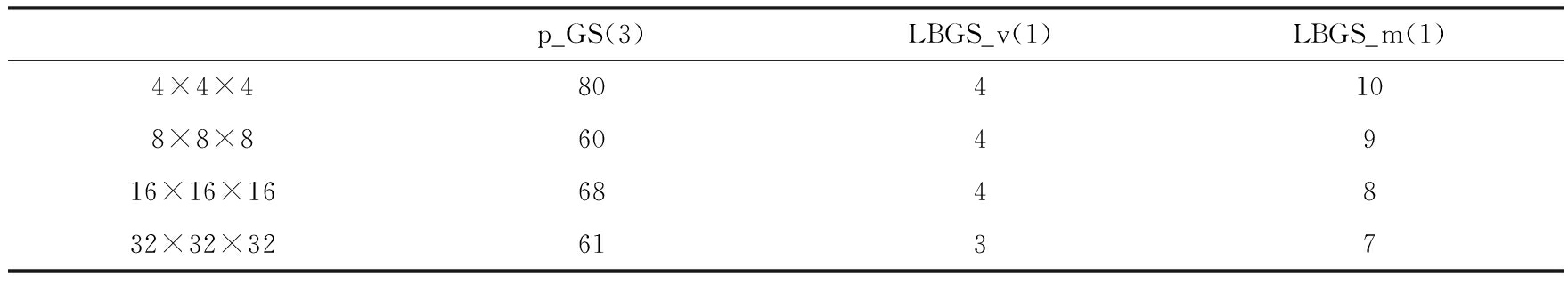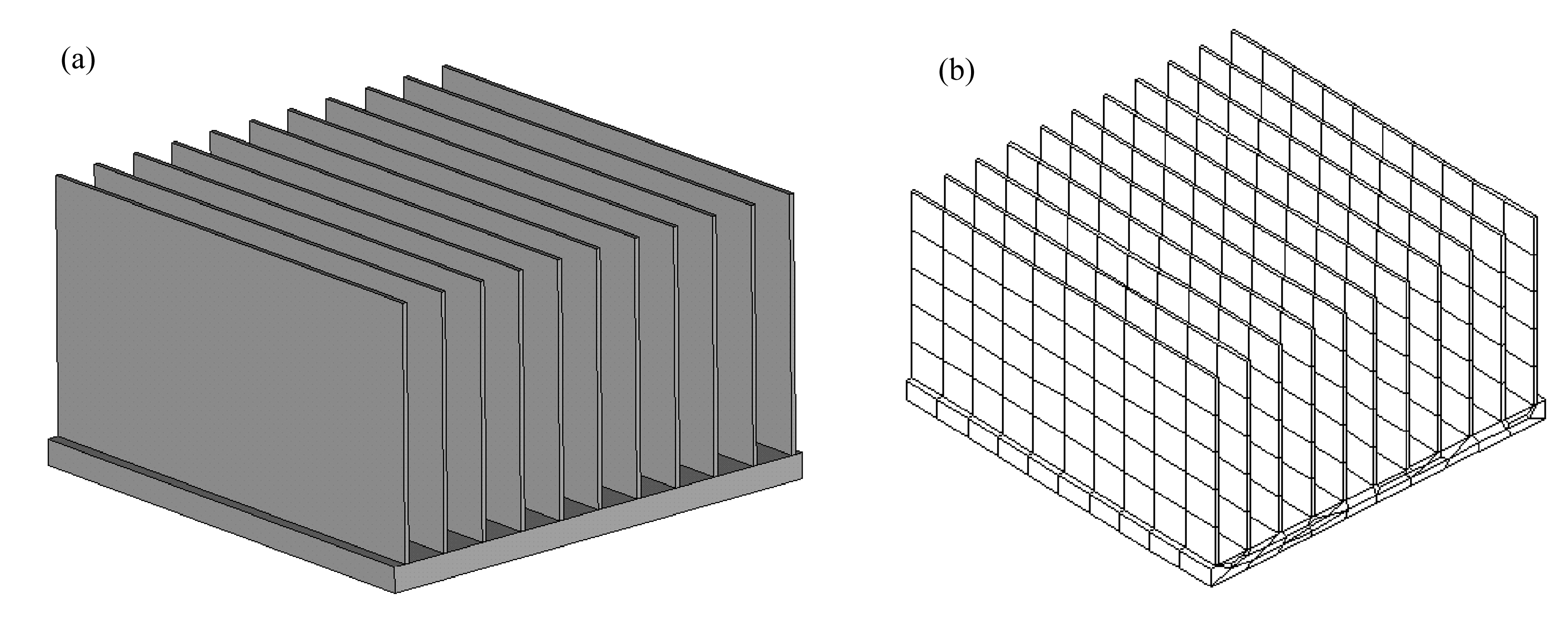Fig. 5 The geometry structure of the radiator and one sample mesh with 990 elements

Table 4 Iteration counts of the HTL methods for the hierarchical quadratic discretizations of the radiator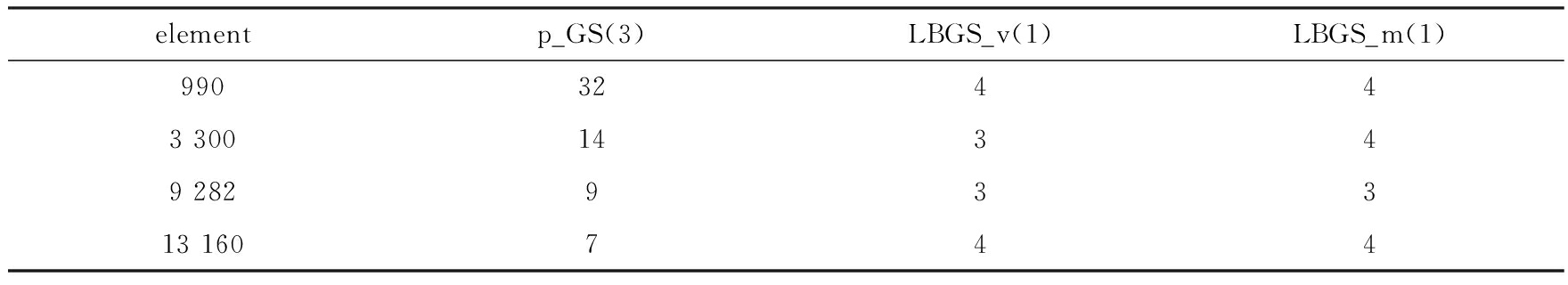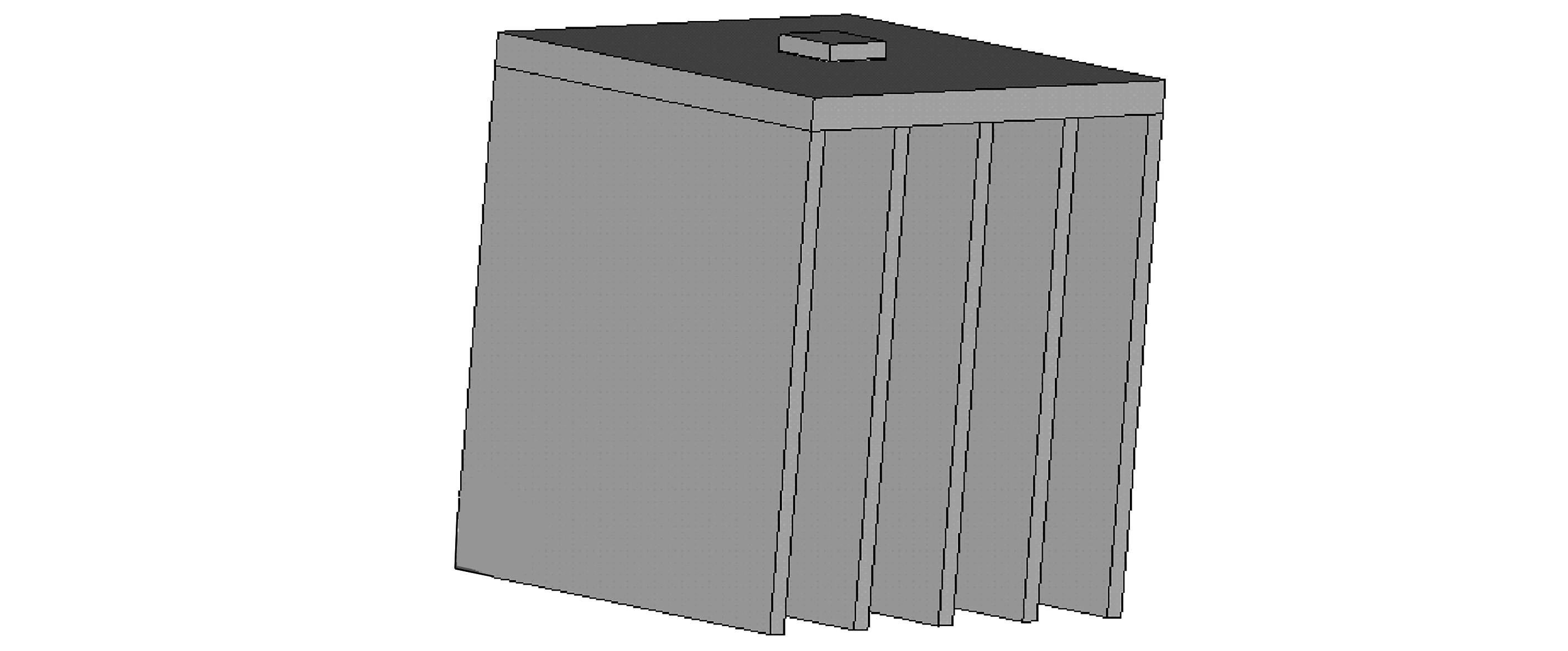Fig. 6 The geometry structure of the LED single chip

Table 5 The sizes and the thermal conductivity of each component of the LED single chip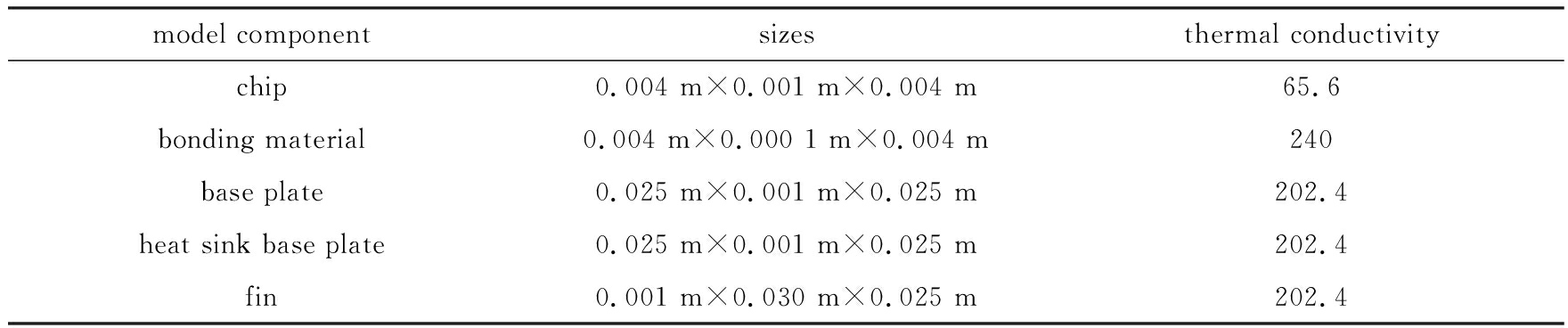Table 6 Iteration counts of the HTL methods for the hierarchical quadratic discretizations of the LED single chip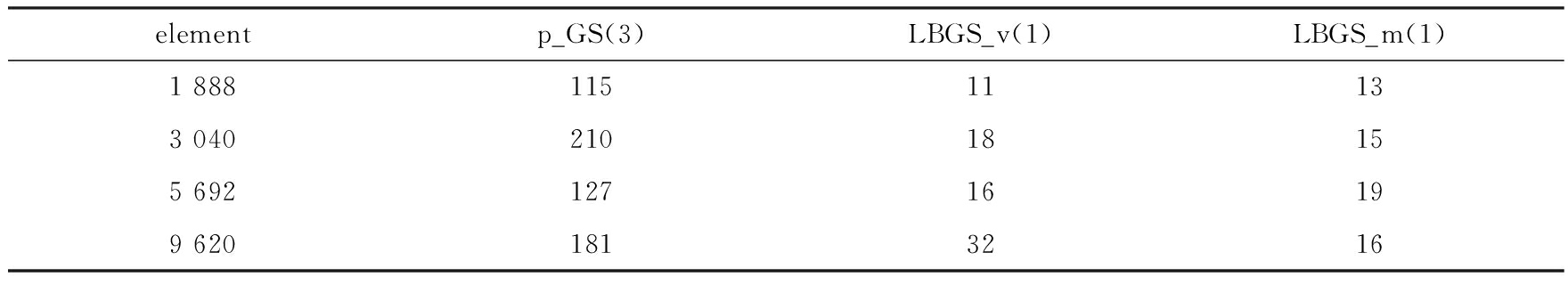1) 对各向同性网格问题，p_GS法具有很好的磨光效果，相应的两水平迭代法求解分层二次有限元方程具有很好的计算效率和鲁棒性例如，对热传导细长梁问题，不管是常系数还是变系数，HTL(p_GS(3))的迭代次数基本不随问题规模的增加而增加

2) 对各向异性网格(薄单元)问题，p_GS法的磨光效果将变差，两种特殊块磨光算子(LBGS_v或LBGS_m)具有更好的磨光效果，相应两水平迭代法的收敛性基本不依赖于问题规模比较而言，基于LBGS_v的两水平迭代法的迭代次数更少，对薄结构热传导问题，在设计块磨光算子时，仅需对所有的顶点在其patches上进行块Gauss-Seidel迭代，以提高运算效率

### 2.2多水平方法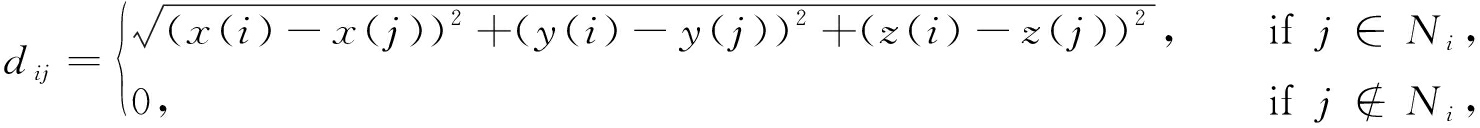(13)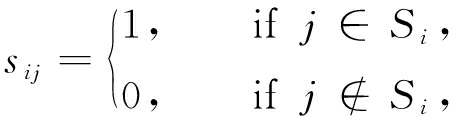(14)

Table 7 The iteration counts and CPU time of several AMG methods for the linear

discretizations of the conduction beam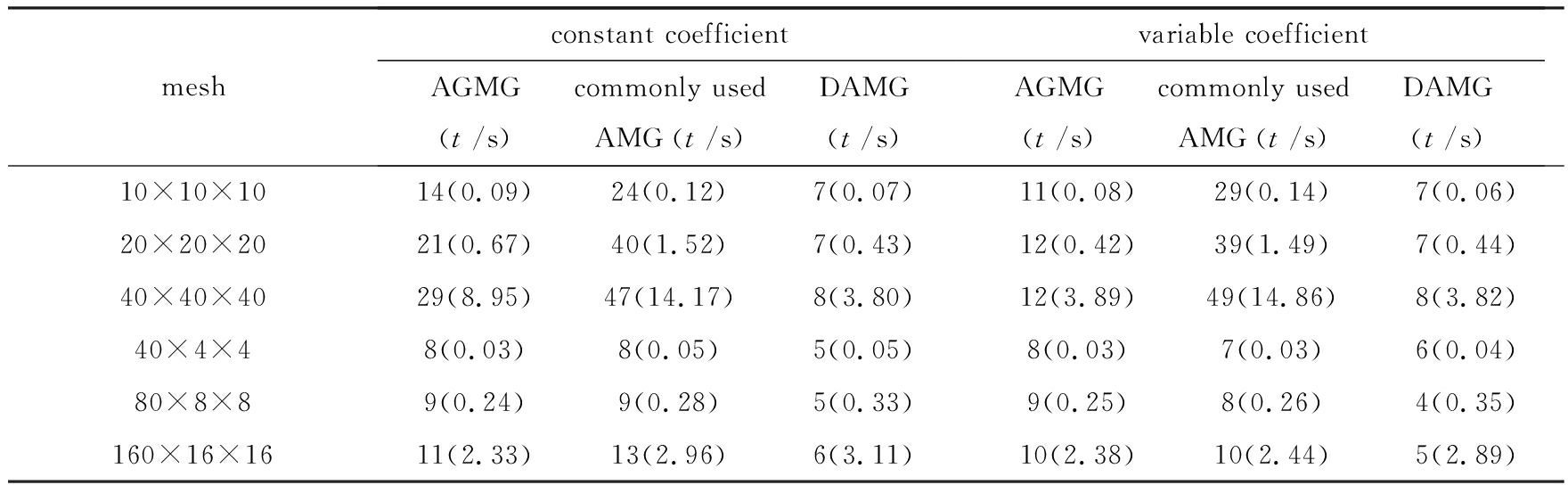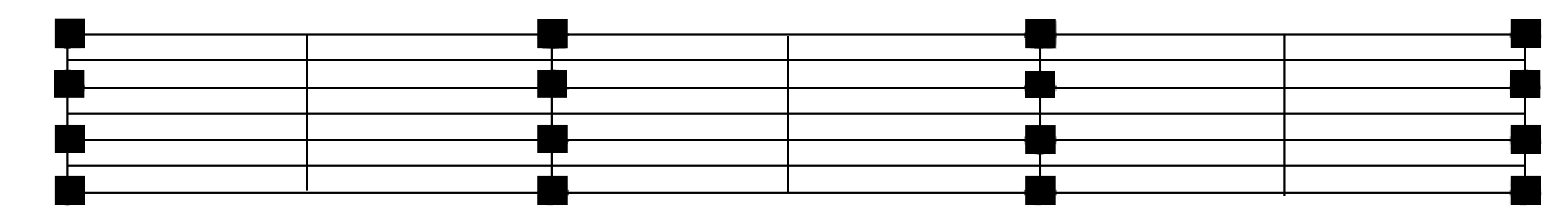(a) 基于MIS粗化方法
(a) The coarse grid selection process based on the MIS method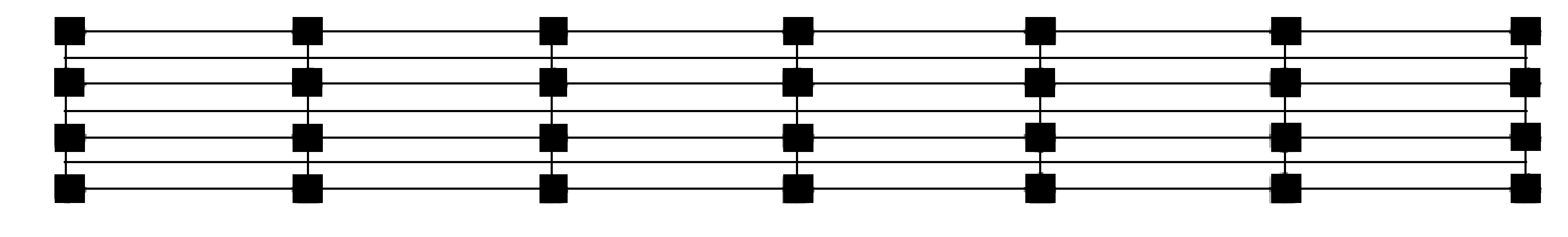(b) 基于“距离矩阵”粗化方法
(b) The coarse grid selection process based on the distance matrix

Fig. 7 Comparison of the results obtained by 2 coarse grid selection processes, where the coarse nodes are denoted by black square and the rest are fine nodes

Table 8 The iteration counts of the HML(S) methods with different smoothers

(a) 热传导细长梁

(a) The case of the slender conduction beam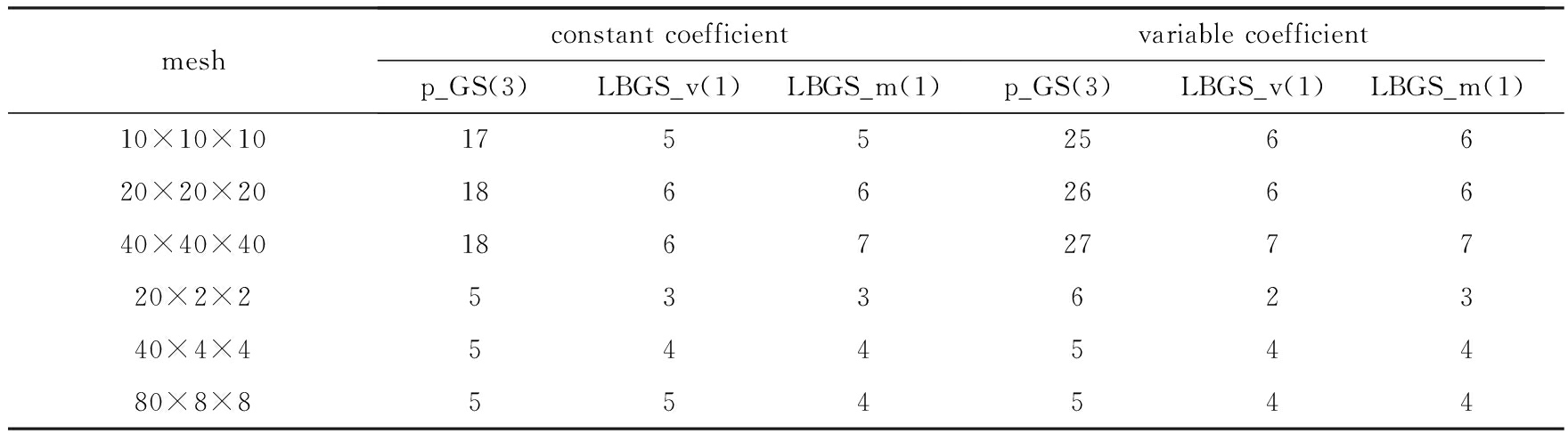(b) 扇形薄结构

(b) The case of the thin-walled sector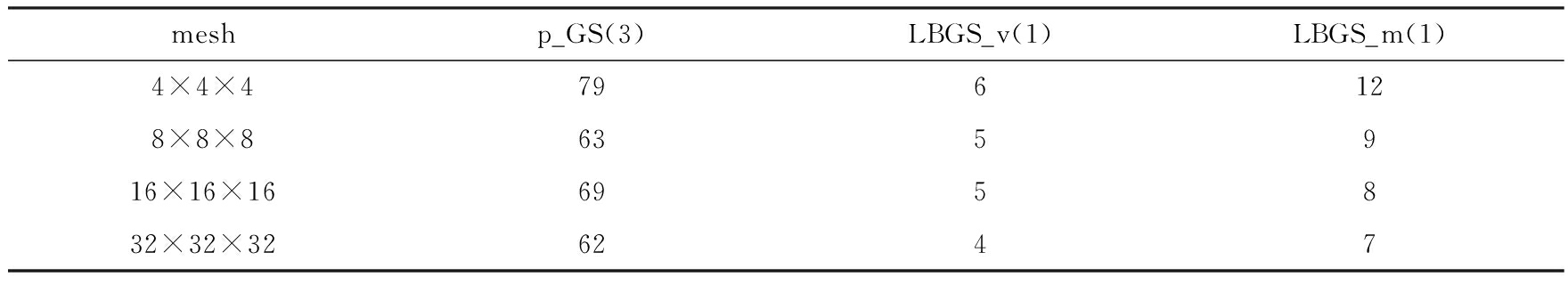(c) 散热片问题

(c) The case of the radiator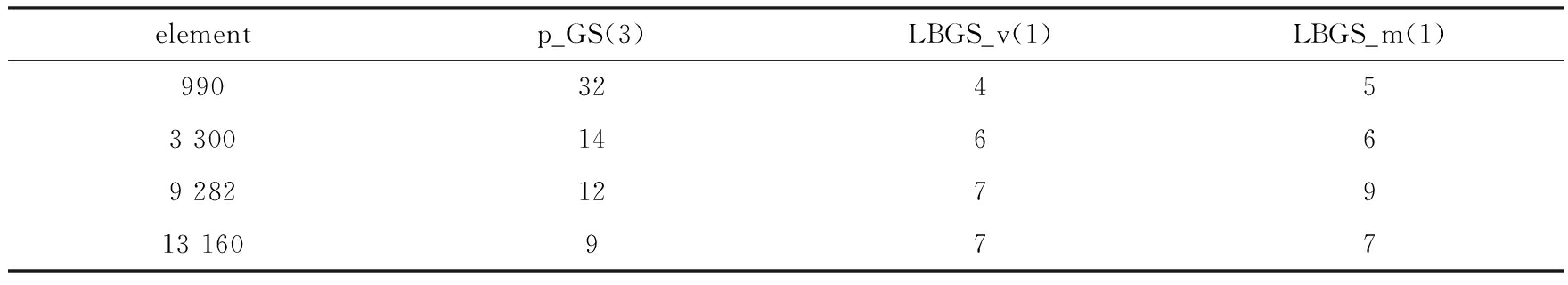(d) LED单芯片

(d) The case of the LED single chip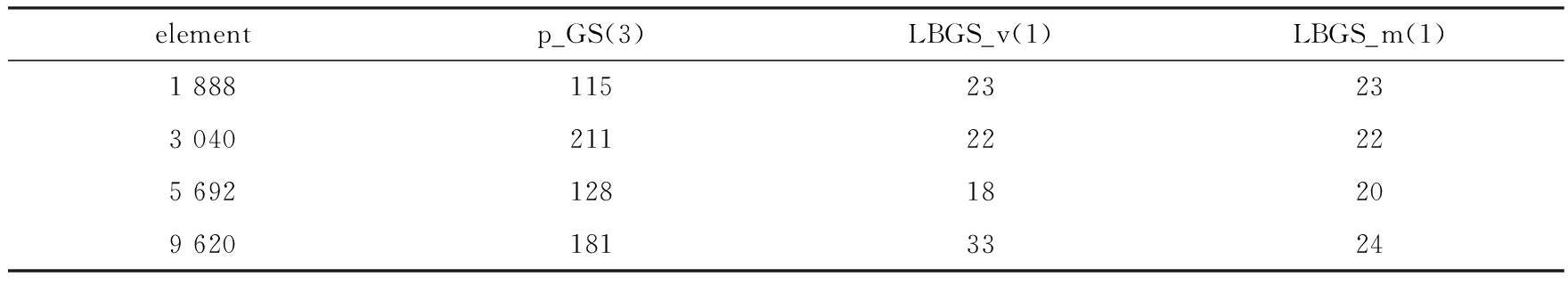Table 9 The iteration counts of the HML(S) methods by calling l times of

DAMG to solve the coarse level discretizations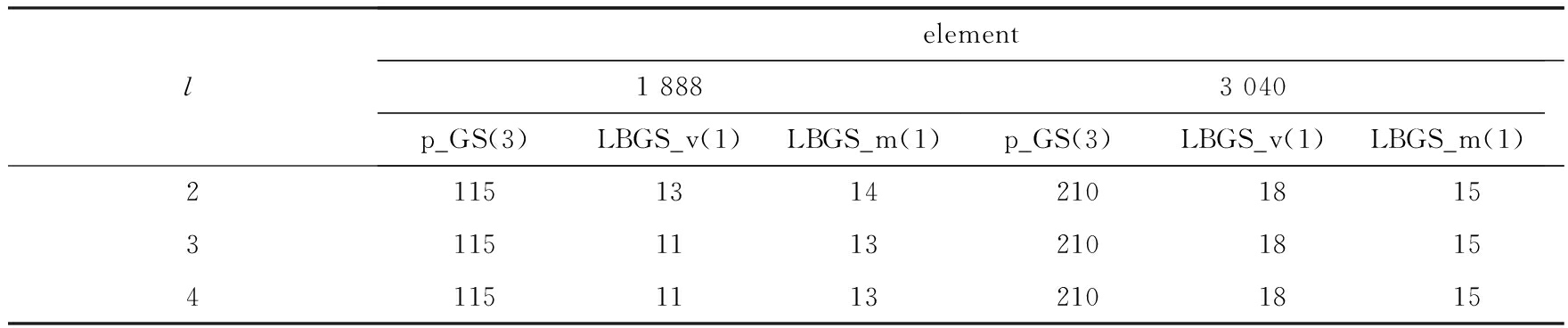## 3 结 语

 BOUZAKIS K D, VIDAKIS N. Prediction of the fatigue behavior of physically vapor deposited coating in the ball-on-rod rolling contact fatigue test, using an elastic-plastic finite elements method simulation[J]. Wear, 1997, 206(1/2): 197-203.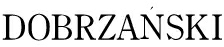L A,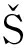LIWA A, KWANY W. Employment of the finite element method for determining stresses in coatings obtained on high-speed steel with the PVD process[J]. Journal of Materials Processing Technology, 2005, 164/165: 1192-1196.

 BRANDT A, MCCORMICK S, RUGE J. Algebraic multigrid (AMG) for sparse matrix equations[C]//Sparsity and Its Applications. Loughborough, 1983: 257-284.

 BREZINA M, CLEARY A J, FALGOUT R D, et al. Algebraic multigrid based on element interpolation (AMGe)[J]. SIAM Journal on Scientific Computing, 2000, 22(5): 1570-1592.

 CLEARY A J, FALGOUT R D, HENSON V E, et al. Robustness and scalability of algebraic multigrid[J]. SIAM Journal on Scientific Computing, 2000, 21(5): 1886-1908.

 GRIEBEL M, OELTZ D, SCHWEITZER M A. An algebraic multigrid method for linear elasticity[J]. SIAM Journal on Scientific Computing, 2003, 25(2): 385-407.

 BAKER A H, KOLEV T V, YANG U M. Improving algebraic multigrid interpolation operators for linear elasticity problems[J]. Numerical Linear Algebra With Applications, 2010, 17(2/3): 495-517.

 SHU Shi, XU Jinchao, YANG Ying, et al. An algebraic multigrid method for finite element systems on criss-cross grids[J]. Advances in Computational Mathematics, 2006, 25(1/3): 287-304.

 VAN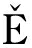K P, MANDEL J, BREZINA M. Algebraic multigrid by smoothed aggregation for second and fourth order elliptic problems[J]. Computing, 1996, 56(3): 179-196.

 NOTAY Y. An aggregation-based algebraic multigrid method[J]. Electronic Transactions on Numerical Analysis, 2010, 37: 123-146.

 RUGE J, MCCORMICK S, MANTEUFFEL T, et al. AMG for higher-order discretizations of second-order elliptic problems[C]//Abstracts of the Eleventh Copper Mountain Conference on Multigrid Methods. 2003.

 HEYS J J, MANTEUFFEL T A, MCCORMICK S F, et al. Algebraic multigrid for higher-order finite elements[J]. Journal of Computational Physics, 2005, 204: 520-532.

 EL MALIKI A, GUÉNETTE R, FORTIN M. An efficient hierarchical preconditioner for quadratic discretizations of finite element problems[J]. Numerical Linear Algebra With Applications, 2011, 18: 789-803.

 SHU S, SUN D, XU J. An algebraic multigrids for higher order finite element discretizations[J]. Computing, 2006, 77(4): 347-377.

 XIAO Yingxiong, SHU Shi. A robust preconditioner for higher order finite element discretizations in linear elasticity[J]. Numerical Linear Algebra With Applications, 2011, 18: 827-842.

 谭敏, 肖映雄, 舒适. 一种各向异性四边形网格下的代数多重网格法[J]. 湘潭大学自然科学学报, 2005, 27(1): 78-84.(TAN Min, XIAO Yingxiong, SHU Shi. An algebraic multigrid method on anisotropic quadrilateral grid[J]. Natural Science Journal of Xiangtan University, 2005, 27(1): 78-84.(in Chinese))

 XIAO Yingxiong, SHU Shi, ZHANG Ping, et al. An algebraic multigrid method for isotropic linear elasticity on anisotropic meshes[J]. International Journal for Numerical Methods in Biomedical Engineering, 2010, 26: 534-553.

 GRAHAM E, FORSYTH P A. Preconditioning methods for very ill-conditioned three-dimensional linear elasticity problems[J]. International Journal for Numerical Methods in Engineering, 1999, 44(1): 77-99.

 SUMANT P S, CANGELLARIS A C, ALURU N R. A node-based agglomeration AMG solver for linear elasticity in thin bodies[J]. Communications in Numerical Methods in Engineering, 2009, 25: 219-236.

 YSERENTANT H. On the multi-level spliting of finite element spaces[J]. Numerische Mathematik, 1986, 49(4): 379-412.

 XIAO Yingxiong, ZHOU Zhiyang, SHU Shi. An efficient AMG method for quadratic discretizations of linear elasticity problems on some typical anisotropic meshes in three dimensions[J]. Numerical Linear Algebra With Applications, 2015, 22(3): 465-482.

 WU S C, LIU G R, ZHANG H O, et al. A node-based smoothed point interpolation method (NS-PIM) for three-dimensional heat transfer problems[J]. International Journal of Thermal Sciences, 2009, 48(7): 1367-1376.

ZHANG Shen, XIAO Yingxiong, GUO Ruiqi. A multi-level method for hierarchical quadratic discretizations of thin-walled structures in 3D heat conduction[J]. Applied Mathematics and Mechanics, 2018, 39(6): 700-713.

# A Multi-Level Method for Hierarchical Quadratic Discretizations of Thin-Walled Structures in 3D Heat Conduction①

ZHANG Shen, XIAO Yingxiong, GUO Ruiqi

(College of Civil Engineering and Mechanics, Xiangtan University, Xiangtan, Hunan 411105, P.R.China)

Abstract: When the finite element method is applied to analyze the 3D thin-walled structures, some thin hexahedral elements are usually used in order to reduce the number of elements, and the corresponding higher-order elements are preferred since they have some obvious advantages in the calculation accuracy, the anti-distortion degree and so on. However, they have much higher computational complexity than the lower-order (e.g., linear) elements and the coefficient matrix of the linear algebraic equation system is severely ill-conditioned. The convergence of the commonly used solvers will deteriorate with the increasing size of the problem. An efficient and robust multi-level method was presented for the hierarchical quadratic discretizations of 3D thin-walled structures through combination of two special local block Gauss-Seidel smoothers and the DAMG algorithm based on the distance matrix. Since a hierarchical basis is used, those algebraic criteria are not needed to judge the relationships between the unknown variables and the geometric node types, and the grid transfer operators are also trivial. This makes it easy to find the coarse level (linear element) matrix derived directly from the fine level matrix, and thus the overall efficiency is greatly improved. The numerical results verify the efficiency and robustness of the proposed method.

Key words: heat conduction problem; thin-walled structure; hierarchical quadratic element; multi-level method; algebraic multigrid

Foundation item: The National Natural Science Foundation of China(11601462)

DOI: 10.21656/1000-0887.380035

*收稿日期 2017-02-16； 修订日期： 2017-11-30

ⓒ 应用数学和力学编委会，ISSN 1000-0887